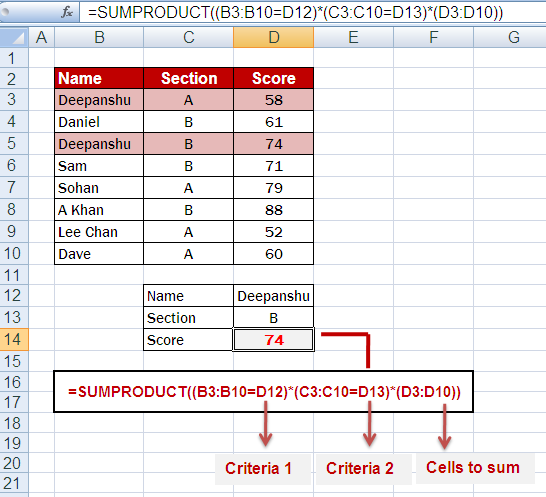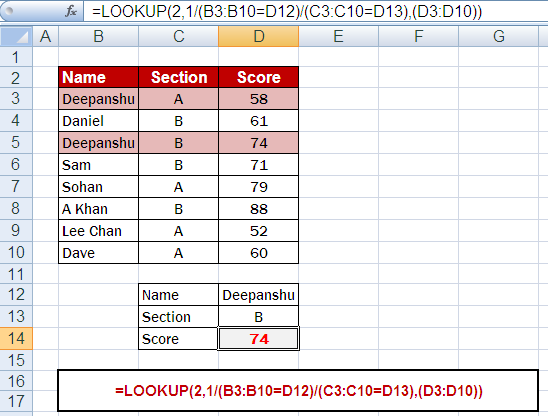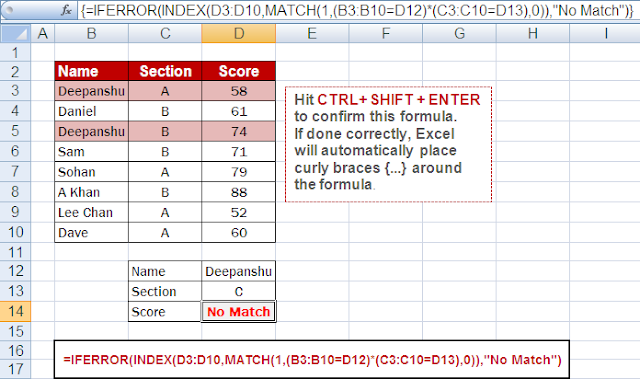# 7 Ways to Lookup Multiple Criteria or Conditions in Excel

This article explains multiple ways to lookup a value based on multiple criteria or conditions in Excel.

Scenario

Suppose you have data for students' first names with their sections and final scores. Two students can have a similar first name in different sections. You are asked to find the score of a student given its name and section.

Sample File
1. SUMPRODUCTFORMULA :
=SUMPRODUCT((B3:B10=D12)*(C3:C10=D13)*(D3:D10))
1. The formula compares each cell in the range B3:B10 with the value in cell D12 (Name). It creates an array of TRUE/FALSE values.
2. Compare each cell in the range C3:C10 with the value in cell D13 (Section), creating another array of TRUE/FALSE values.
3. Keep the values in the range D3:D10 as they are.
4. Multiply the corresponding elements of all three arrays together, considering only the cells where both conditions (matching values in B3:B10 and C3:C10) are satisfied.
5. Sum up all the products obtained in the previous step, resulting in the desired output i.e. score of a student.
2. SUM (ARRAY FORMULA)FORMULA :
=SUM((B3:B10=D12)*(C3:C10=D13)*(D3:D10))

Hit CTRL+ SHIFT + ENTER to confirm this formula. If done correctly, Excel will automatically place curly braces {...} around the formula.

After placing curly braces, the formula would look like this in formula bar :
{=SUM((B3:B10=D12)*(C3:C10=D13)*(D3:D10))}
3. SUMIFSFORMULA :
=SUMIFS(D3:D10,B3:B10,D12,C3:C10,D13)

The formula calculates the sum of the values in the range D3:D10 that meet multiple criteria. It adds up the values from D3:D10 where the corresponding cells in B3:B10 match the value in D12 and the corresponding cells in C3:C10 match the value in D13.

4. INDEX-MATCHFORMULA :
=INDEX(D3:D10,MATCH(1,(B3:B10=D12)*(C3:C10=D13),0))
Hit CTRL+ SHIFT + ENTER to confirm this formula. If done correctly, Excel will automatically place curly braces {...} around the formula.
After placing curly braces, the formula would look like this in formula bar :
{=INDEX(D3:D10,MATCH(1,(B3:B10=D12)*(C3:C10=D13),0))}

The formula fetches the value from the range D3:D10 that meets the criteria specified. It uses the MATCH function to find the position of the first occurrence of "1" in the array created by multiplying (B3:B10=D12) and (C3:C10=D13). The INDEX function then returns the corresponding value from the range D3:D10 at that position.

5. INDEX-MATCH (Version 2)FORMULA :
=INDEX(D3:D10,MATCH(1,IF(B3:B10=D12,IF(C3:C10=D13,1)),0))
Hit CTRL+ SHIFT + ENTER to confirm this formula. If done correctly, Excel will automatically place curly braces {...} around the formula.
After placing curly braces, the formula would look like this in formula bar :
{=INDEX(D3:D10,MATCH(1,IF(B3:B10=D12,IF(C3:C10=D13,1)),0))}
6. LOOKUPFORMULA :
=LOOKUP(2,1/(B3:B10=D12)/(C3:C10=D13),(D3:D10))

The formula searches for the value "2" in the array created by dividing 1 by the logical conditions (B3:B10=D12) and (C3:C10=D13). It then returns the corresponding value from the range D3:D10. This formula essentially performs a reverse lookup to find the last value that matches the specified criteria.

7. IF NO MATCH FOUNDFORMULA :
=IFERROR(INDEX(D3:D10,MATCH(1,(B3:B10=D12)*(C3:C10=D13),0)),"No Match")
This formula returns "No Match" if no value exists on the basis of conditions. Hit CTRL+ SHIFT + ENTER to confirm this formula. If done correctly, Excel will automatically place curly braces {...} around the formula.

After placing curly braces, the formula would look like this in formula bar : {=IFERROR(INDEX(D3:D10,MATCH(1,(B3:B10=D12)*(C3:C10=D13),0)),"No Match")}
Related Posts
ShareDeepanshu founded ListenData with a simple objective - Make analytics easy to understand and follow. He has over 10 years of experience in data science. During his tenure, he worked with global clients in various domains like Banking, Insurance, Private Equity, Telecom and HR.

4 Responses to "7 Ways to Lookup Multiple Criteria or Conditions in Excel"
1.WHAT IF STUDENT BELONG FROM SAME SECTION AND SAME FIRST NAME.?

2.PREMLATA2733@GMAIL.COM
PROVIDE THE EXCEL SHEET

3.Thank you! What a big help!

4.The explanation of 4. INDEX-MATCH was exactly what I was looking for - works great when comparing 2 conditions in different worksheets and returning a third value

Next → ← Prev
Looks like you are using an ad blocker!

To continue reading you need to turnoff adblocker and refresh the page. We rely on advertising to help fund our site. Please whitelist us if you enjoy our content.